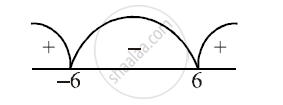ISC (Commerce) Class 12CISCE
Share

# The Average Cost Function, Ac for a Commodity is Given by Ac = X + 5 + 36/X in Terms of Output X. Find the Total Cost, C and Marginal Cost, Mc as a Function of X. The Outputs for Which Ac Increases - ISC (Commerce) Class 12 - Mathematics

ConceptApplication of Calculus in Commerce and Economics in the Average Cost

#### Question

The average cost function, AC for a commodity is given by AC = x + 5 + 36/x in terms of output x. Find

1) The total cost, C and marginal cost, MC as a function of x.

2) The outputs for which AC increases

#### Solution

Let C be the total cost function. Then

Average cost (AC) = C/x => AC.x => C = (x + 5 + 36/x) x

=> C = x^2 + 5x + 36

Let MC be the marginal cost function. Then,

MC = (dC)/dx = d/dx (x^2 + 5x + 36) = 2x + 5

Now, d/dx (AC) = d/dx (x + 5 + 36/x) = 1 - 36/x^2

For AC to be increasing d/dx(AC) > 0 => 1 - 36/x^2 > 0

=> x^2 - 36 > 0 => (x - 6) (x + 6) > 0

=> x < -6 or x > 6 => x > 6    [∵ x > 0]Is there an error in this question or solution?

#### APPEARS IN

2014-2015 (March) (with solutions)
Question 14.2 | 5.00 marks
Solution The Average Cost Function, Ac for a Commodity is Given by Ac = X + 5 + 36/X in Terms of Output X. Find the Total Cost, C and Marginal Cost, Mc as a Function of X. The Outputs for Which Ac Increases Concept: Application of Calculus in Commerce and Economics in the Average Cost.
S